# Frank solutions for ICSE Class 10 Physics Part 2 chapter 4 - Current Electricity [Latest edition]

#### Chapters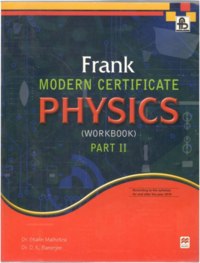## Chapter 4: Current Electricity

Exercise 4.1ExerciseExercise 4.2Exercise 4.3
Exercise 4.1, Exercise [Pages 173 - 213]

### Frank solutions for ICSE Class 10 Physics Part 2 Chapter 4 Current Electricity Exercise 4.1, Exercise [Pages 173 - 213]

Exercise 4.1 | Q 1 | Page 173
What do you mean by a cell?
Exercise 4.1 | Q 2 | Page 173
What is the difference between a cell and a battery?
Exercise 4.1 | Q 3 | Page 173
What do you understand by an electric circuit?
Exercise 4.1 | Q 4 | Page 173
Name any four components of an electric circuit. Write their symbols also.
Exercise 4.1 | Q 5 | Page 173
What do you mean by a circuit diagram?
Exercise 4.1 | Q 6 | Page 173

What is wrong with the circuit diagram (Fig. 34)? Carefully check the symbols and assign proper positive and negative signs to them.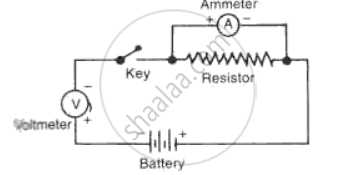Exercise 4.1 | Q 7 | Page 173
State Ohm's law. Is it always true?
Exercise 4.1 | Q 8 | Page 173
What is electrical resistance? Write its SI unit.
Exercise 4.1 | Q 9 | Page 173
Name the physical quantity that represents the ratio of potential difference and current.
Exercise 4.1 | Q 10 | Page 173
Define one ohm.
Exercise 4.1 | Q 11 | Page 173
Does Ohm's law hold good for semiconductors and electrolytic solutions?
Exercise 4.1 | Q 12 | Page 173
State the factors on which the resistance of a conductor depends.
Exercise 4.1 | Q 13 | Page 173
What do you mean by an equivalent resistance?
Exercise 4.1 | Q 14 | Page 173

A simple electric circuit has 24 V battery and a resistor of 60 Ω. What will be the current in the circuit? The resistance of the connecting wires is negligible.

Exercise 4.1 | Q 15 | Page 173

A graph is plotted taking p.d. along y-axis and electric current along x-axis. Name the physical quantity that represents the slope of this graph (Fig.).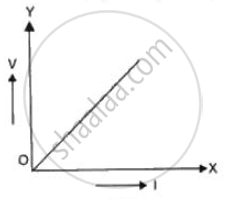Exercise 4.1 | Q 16 | Page 173
Define resistivity of a metal.
Exercise 4.1 | Q 17 | Page 173
What are the SI units of specific resistance?
Exercise 4.1 | Q 18 | Page 173
What do you mean by conductance of a conductor?
Exercise 4.1 | Q 19 | Page 173

Name the following substances:
(i) Showing low resistivity,
(ii) Showing very high resistivity,
(iii) Showing moderate resistivity.

Exercise 4.1 | Q 20 | Page 173

What is the current I in the given circuit (Fig. )?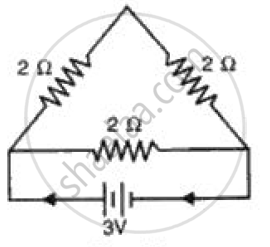Exercise | Q 49 | Page 213

What is the function of a step-up transformer?

Exercise 4.1 [Page 174]

### Frank solutions for ICSE Class 10 Physics Part 2 Chapter 4 Current Electricity Exercise 4.1 [Page 174]

Exercise 4.1 | Q 1 | Page 174
State the law which relates the current in a conductor to the potential difference across its ends.
Exercise 4.1 | Q 2 | Page 174
What happens to the resistance if the conductor is made thinner?
Exercise 4.1 | Q 3 | Page 174
What kind of plot would you except when current (I) is plotted against potential difference (V) for a conductor, at constant temperature?
Exercise 4.1 | Q 4 | Page 174
What is an ammeter? How is it connected in a circuit?
Exercise 4.1 | Q 5 | Page 174
What is a voltmeter? How is it connected in a circuit?
Exercise 4.1 | Q 6 | Page 174
Does an ammeter have a low or high resistance?
Exercise 4.1 | Q 7 | Page 174
Does a voltmeter have a low or high resistance?
Exercise 4.1 | Q 8 | Page 174
What is the role of a key in an electric circuit?
Exercise 4.1 | Q 9 | Page 174
What do you mean by a rheostat?
Exercise 4.1 | Q 10 | Page 174

A simple electric circuit has a 30 V battery and a resistor ot 60 o. What will be the current in the circuit? The resistance of the oonnecting wires is negligible.

Exercise 4.1 | Q 11 | Page 174
Potential difference between two points of a wire carrying 2 ampere current is 0.1 volt. Calculate the resistance between these points.
Exercise 4.1 | Q 12 | Page 174
The potential difference between the terminals of an electric iron is 240 V and the current flowing through it is 6 A. What is the resistance of the electric iron?
Exercise 4.1 | Q 13 | Page 174

What is the current in the circuit shown (Fig. )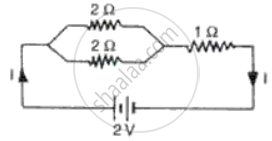Exercise 4.1 | Q 14 | Page 174

What potential difference is needed to drive a current o f 1 A through a 5 Ω resistor?

Exercise 4.1 | Q 15 | Page 174

A potential difference of 6 V is applied across a 3 Ω resistor. What is the current flowinq throuqh the circuit?

Exercise 4.1 | Q 16 | Page 174
What is the value of a resistance if 20 V drives 2 A through it?
Exercise 4.1 | Q 17 | Page 174

What is the equivalent resistance between A and B in the given circuit (Fig?)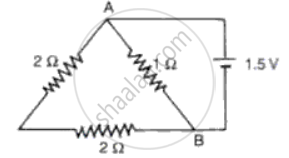Exercise 4.1 | Q 18 | Page 174

What is the equivalent resistance of 5 equal resistors, each of value 2Omega 1 when oonnected in (a) Series  ( b) ParaIleI?

Exercise 4.1 | Q 19 | Page 174

How will you oonnect five resistors, each of the value one ohm, to obtain an equivalent resistance of 0.2 Omega

Exercise 4.1 | Q 20 | Page 174
Give two uses of conductors.
Exercise 4.1 [Page 175]

### Frank solutions for ICSE Class 10 Physics Part 2 Chapter 4 Current Electricity Exercise 4.1 [Page 175]

Exercise 4.1 | Q 1 | Page 175

Write the SI units for:
(a) Electric current

(b) Potential difference

(c) Charge.

Exercise 4.1 | Q 2 | Page 175
Define potential difference between two points.
Exercise 4.1 | Q 3 | Page 175
Is electric potential a scalar or a vector physical quantity?
Exercise 4.1 | Q 4 | Page 175
Define electric intensity. Write its SI unit. Is it a scalar or a vector physical quantity?
Exercise 4.1 | Q 5 | Page 175
By what name is the unit joule/coulomb called?
Exercise 4.1 | Q 6 | Page 175
How much work is done when 5 C charge moves across a p.d. of 1 V?
Exercise 4.1 | Q 7 | Page 175

The work done in moving unit positive charge across two points in an electric circuit is a measure of

•  Potential difference

•  Resistance

• Power

• Current

Exercise 4.1 | Q 8 | Page 175

The device used for measuring potential difference is

• Potentiometer

•  Ammeter

• Galvanometer

• Voltmeter

Exercise 4.1 | Q 9 | Page 175
What are the conditions under which charges can move in a conductor?
Exercise 4.1 | Q 10 | Page 175
How will you maintain a potential difference between the ends of a conductor?
Exercise 4.1 | Q 11 | Page 175
What do you mean by electric current?
Exercise 4.1 | Q 12 | Page 175
What is the direction of electric current?
Exercise 4.1 | Q 13 | Page 175
Name the physical quantity which defines the rate of flow of charge.
Exercise 4.1 | Q 14 | Page 175
Name the physical quantity which is measured by an ammeter.
Exercise 4.1 | Q 15 | Page 175
How many electrons are present in 1 C?
Exercise 4.1 | Q 16 | Page 175

A battery of e.m.f. 15 V and internal resistance 3 ohm is connected to two resistors of resistance 3 ohm and 6 ohm in series. Find:
(i) The current through the battery,
(ii) The p.d. between the terminals of the battery.

Exercise 4.1 | Q 17 | Page 175

A wire of uniform thickness with a resistance of 27 O is cut into three equal pieces and they are joined in parallel. Find the resistance of the parallel combination.

Exercise 4.1 | Q 18 | Page 175

You have three resistors of values 2 Omega, 3 Omega, and 5 Omega. How will you join them so that the total resistance is less than 2 Omega?

Exercise 4.1 | Q 19 | Page 175

Calculate the equivalent resistance of the following combination of resistor r1, r2, r3, and r4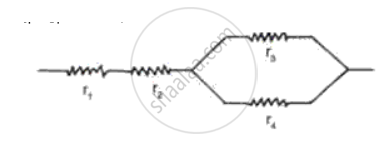Exercise 4.1 | Q 20 | Page 175
A combination consists of three resistors in series. Four similar sets are connected in parallel. If the resistance of each resistor is 2 ohm, find the resistance of the combination.
Exercise 4.2 [Page 187]

### Frank solutions for ICSE Class 10 Physics Part 2 Chapter 4 Current Electricity Exercise 4.2 [Page 187]

Exercise 4.2 | Q 1 | Page 187
Define electric power. What do you mean by 'power rating' of an electric appliance?
Exercise 4.2 | Q 2 | Page 187
Distinguish between electric power and electrical energy. State their units. Give the other name of kWh.
Exercise 4.2 | Q 3 | Page 187

Fig. represents the circuit used for the verification of ohm's law. Label the different parts from A and F. State the function of each.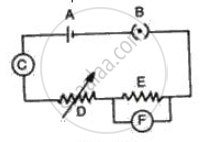Exercise 4.2 | Q 4 | Page 187
Explain the meaning of the statement 'the current rating of a fuse is 5 A'.
Exercise 4.2 | Q 5 | Page 187
'A fuse is rated 8 A'. Can it be used with an electrical appliance of rating 5 kW, 200 V?
Exercise 4.2 | Q 6 | Page 187
An electric kettle is rated 3 kW, 250 V. Give reason whether this kettle can be used in a circuit which contains a 13 A fuse.
Exercise 4.2 | Q 7 | Page 187
Calculate the electric current supplied by a 12 V battery of an automobile to a starter that develops a power of 2.4 kW.
Exercise 4.2 | Q 8 | Page 187

A 720 W heater operates from a 220 V line voltage. Calculate:
(a) The electrical energy consumed by the heater in 3 h.
(b) The cost of electrical energy consumption at the rate of 60 paise per kWh.

Exercise 4.2 | Q 9 | Page 187
What is fuse? Name the material of fuse.
Exercise 4.2 | Q 10 | Page 187
State two characteristics of a fuse wire.
Exercise 4.2 | Q 11.1 | Page 187

Complete the following sentence:
A fuse is a short piece of wire of high ......... and low

Exercise 4.2 | Q 11.2 | Page 187

Complete the following sentence:

Fuse wire has a ............ Melting point and it is made of an alloy of ......... and if the current in a circuit rises too high, the fuse wire ...........

Exercise 4.2 | Q 11.3 | Page 187

Complete the following sentence:

A fuse is connected in ........... with the .........  wire.

Exercise 4.2 [Pages 188 - 199]

### Frank solutions for ICSE Class 10 Physics Part 2 Chapter 4 Current Electricity Exercise 4.2 [Pages 188 - 199]

Exercise 4.2 | Q 1 | Page 188

Calculate the amount of energy transferred by a 10 A electric current flowing through a resistor of 5 o for 15 minutes.

Exercise 4.2 | Q 2 | Page 188
A 200 v electric transfer draws an electric current of 11 A. What is its electrical resistance?
Exercise 4.2 | Q 3 | Page 188
A heater is rated at 1200 W when the electric current is 5 A. Calculate the resistance and the potential difference.
Exercise 4.2 | Q 4 | Page 188
Where is the main fuse in a house circuit connected?
Exercise 4.2 | Q 5 | Page 188
Is kWh the unit of electrical energy?
Exercise 4.2 | Q 6 | Page 188
What is the purpose of a switch in a circuit? Why is the switch put in the live wire?
Exercise 4.2 | Q 7 | Page 188

A switch is not touched with wet hands while putting it on or off. Give a reason for your answer.

Exercise 4.2 | Q 8 | Page 188
An electric bulb is rated 250 W, 230 V. What information does this convey? Calculate the current through a 60 W lamp rated for 250 V. If the line voltage falls to 200 V, how is the power consumed by the bulb affected?
Exercise 4.2 | Q 9 | Page 188
The metal case of an electric appliance is earthed. Explain the reason.
Exercise 4.2 | Q 10 | Page 188

A bulb marked 12 V, 24 W operates on a 12 volt car battery for 20 minutes. Calculate:
(i) The current flowing through it, and
(ii) The energy liberated.

Exercise 4.2 | Q 11 | Page 188

What is international convention of colour coding in a cable?

Exercise 4.2 | Q 12 | Page 188

A current of 0.2 A flows through a wire whose ends are at a potential difference of 15 V. Calculate:
(i) The resistance of the wire, and
(ii) The heat energy produced in 1 minute.

Exercise 4.2 | Q 13 | Page 188
What should be the current rating of a fuse to be used with an electric appliance of rating 1.5 kW-220 V? How is the fuse connected with the appliance?
Exercise 4.2 | Q 14 | Page 188
Explain briefly how a fuse protects an electric circuit.
Exercise 4.2 | Q 15 | Page 188
Why is earthing done?
Exercise 4.2 | Q 16 | Page 188
Draw a labeled diagram of a three pin socket.
Exercise 4.2 | Q 17 | Page 188
Under what circumstances does one get an electric shock from an electrical gadget?
Exercise 4.2 | Q 18 | Page 188

State the S.I. units of electrical power and electrical energy.
(i) Define the household unit of electricity.
(ii) What is the voltage of the electricity that is generally supplied to a house?

Exercise 4.2 | Q 19 | Page 188
An electric bulb is rated at 220 V, 100 W. What is its resistance? What maximum current can be passed through it?
Exercise 4.2 | Q 20 | Page 188

Two resistors of 4 Ω and 6 Ω are connected in parallel. The combination is
connected across a 6 volt battery of negligible resistance. Calculate:
( i) The power supplied by the battery,
(ii) The power dissipated in each resistor.

Exercise 4.2 | Q 21 | Page 188
What purpose is served by the terminals of a three way pin plug?
Exercise 4.2 | Q 22 | Page 188
Name the unit which a consumer pays as the cost of electricity.
Exercise 4.2 | Q 23 | Page 188

A battery of e.m.f. 15 V and internal resistance 2 Omega is connected to tvvo resistors of 4 ohm and 6 ohm joined.
(i) In series,
(ii) In para 1 lel. Find in each case the electrica I energy spent per minute in 6 ohm resistor.

Exercise 4.2 | Q 24 | Page 199
State the commercial unit of electrical energy.
Exercise 4.2 | Q 25 | Page 188
Write different expressions for electric power (P), using V, I and R.
Exercise 4.2 | Q 26 | Page 188
What do you mean by 'power-voltage' rating of electrical appliances?
Exercise 4.2 | Q 27 | Page 188
Name the physical quantity which equals the product of voltage and current.
Exercise 4.2 | Q 28 | Page 188
The marking on an electric bulb reads; 200 V, 60 W. What does it mean?
Exercise 4.2 | Q 29 | Page 188
The resistance of 100 W (220 V) bulb is greater than that of 60 W (220 V) bulb. Is it true or false?
Exercise 4.2 [Page 189]

### Frank solutions for ICSE Class 10 Physics Part 2 Chapter 4 Current Electricity Exercise 4.2 [Page 189]

Exercise 4.2 | Q 1 | Page 189
What will be the current drawn by an electric bulb of 40 W when it is connected to a source of 220 V?
Exercise 4.2 | Q 2 | Page 189
What do you mean by watt-hour?
Exercise 4.2 | Q 3 | Page 189
Name the commercial unit of electrical energy.
Exercise 4.2 | Q 4 | Page 189
A radio set of 60 W runs for 50 hours. How much electrical energy is consumed? Give your answer in kWh.
Exercise 4.2 | Q 5 | Page 189
In a house two 60 W electric bulbs are lighted for 4 hours, and three 100 W bulbs for 5 hours every day. How much is the electric energy consumed during the month of June?
Exercise 4.3 [Page 205]

### Frank solutions for ICSE Class 10 Physics Part 2 Chapter 4 Current Electricity Exercise 4.3 [Page 205]

Exercise 4.3 | Q 1 | Page 205
Can you produce electricity from magnetism?
Exercise 4.3 | Q 2 | Page 205
What is electromagnetic induction?
Exercise 4.3 | Q 3 | Page 205
State Fleming's right hand rule.
Exercise 4.3 | Q 4 | Page 205

A coil of copper wire is connected to a galvanometer. What would happen if a bar magnet is:
(i) Pushed into the coil with its north pole entering first?
(ii) Held at rest inside the coil?
(iii) Pulled out again?

Exercise 4.3 | Q 5 | Page 205
When do you use (i) Fleming's left hand rule, and (ii) Fleming's right hand rule?
Exercise 4.3 | Q 6 | Page 205
Can you find the magnitude of current using Fleming's right hand rule?
Exercise 4.3 [Page 206]

### Frank solutions for ICSE Class 10 Physics Part 2 Chapter 4 Current Electricity Exercise 4.3 [Page 206]

Exercise 4.3 | Q 1 | Page 206
What do you mean by direct current?
Exercise 4.3 | Q 2 | Page 206
What do you mean by A.C.?
Exercise 4.3 | Q 3 | Page 206
What is the frequency of D.C.?
Exercise 4.3 | Q 4 | Page 206
What is the frequency of A.C. used in India?
Exercise 4.3 | Q 5 | Page 206
How does an alternating current differ from the direct current?
Exercise 4.3 | Q 6 | Page 206
Which type of current does a generator with commutator produce?
Exercise 4.3 | Q 7 | Page 206
A dynamo converts one from of energy into another. Name the two forms of energy.
Exercise 4.3 | Q 8 | Page 206
In household circuits, is a fuse connected in series or in parallel?
Exercise 4.3 | Q 9 | Page 206
Usually three insulated wires of different colours are used in an electrical appliance. Name the three colours.
Exercise 4.3 | Q 10 | Page 206
What do you mean by short-circuiting?
Exercise 4.3 | Q 11 | Page 206
State two important precautions you should observe while using electricity?
Exercise 4.3 | Q 12 | Page 206
What do you mean by earthing?
Exercise 4.3 | Q 13 | Page 206
What do you mean by an electric fuse?
Exercise 4.3 | Q 14 | Page 206
What is the usual colour code followed for connecting live, neutral and earth wires?
Exercise 4.3 | Q 15 | Page 206
Many electrical appliances and circuits are earthed grounded. What is the reason for this?
Exercise 4.3 | Q 16 | Page 206
Are switches connected in the neutral or live wire?
Exercise 4.3 | Q 17 | Page 206
State Fleming's right hand rule.
Exercise 4.3 | Q 18 | Page 206

State Fleming's left hand rule.

Exercise 4.3 | Q 19 | Page 206
What do you mean by a solenoid?
Exercise 4.3 | Q 20 | Page 206
Can a copper wire be used as a fuse wire?
Exercise 4.3 | Q 21 | Page 206
What type of electricity is supplied to us at houses?
Exercise 4.3 | Q 22 | Page 206
Name the material used for making fuse wire.
Exercise 4.3 | Q 23 | Page 206
What do you mean by earthing?
Exercise 4.3 | Q 24 | Page 206

The diagram shows two ways of connecting three lamps P, Q and R to A.C. supply of 220 V.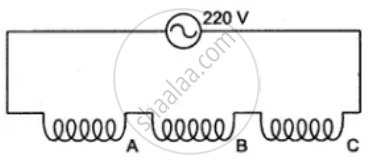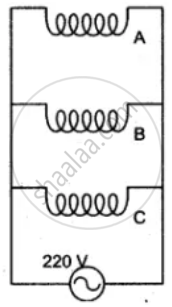Name the two arrangements. Which of them would you prefer in a household circuit? Give a reason for your answer.
Exercise 4.3 | Q 25 | Page 206

Fig shows a three pin plug top. Label the three pins. Why is the top pin thicker and longer than the other two? Why are the pins splitted at the ends?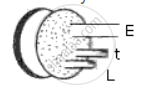Exercise 4.3 | Q 26 | Page 206

The diagram 31 shows a 3 terminal plug socket.
(i) What is the purpose of the terminal E?
(ii) To which part of the appliance is the terminal E connected?
(iii) To which wire L or N, is the fuse connected and why?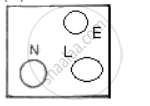Exercise [Page 209]

### Frank solutions for ICSE Class 10 Physics Part 2 Chapter 4 Current Electricity Exercise [Page 209]

Exercise | Q 1.1 | Page 209

Tick(✓) the correct choice in the following:
In the circuit shown in the following fig, the e.m.f. of the cell is 4 volts, the current
flowing through the resistor is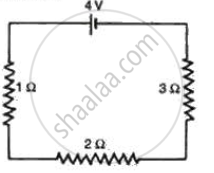• 2/3A

• 2 A

• 11/2 A

• 11/3 A

• 4A

Exercise | Q 1.2 | Page 209

Tick(✓) the correct choice in the following:

When the length of a conductor is doubled the resistance of the conductor
will

• Remain same

• Double in value

• Become four times

• Become one fourth

• Become half

Exercise | Q 1.3 | Page 209

Tick(✓) the correct choice in the following:

Ohm's law is applicable to

• Discharge of electricity through gases

• Diode value

• All metallic conductors

• Carbon compounds

Exercise | Q 1.4 | Page 209

Tick(✓) the correct choice in the following:

24 watt, 12 volt head lamp is fully lit by connecting it to a 12 volt
battery. The working resistance of the lamp is

• 1 Ω

Exercise | Q 1.5 | Page 209

Tick(✓) the correct choice in the following:

Which of the following quantities has the same value for all electrical
appliances connected to the ring main circuit in a house?

• Power

• Voltage

• Resistance

• Current

• Energy

Exercise | Q 1.6 | Page 209

Tick(✓) the correct choice in the following:

The outer casing of an electric iron is generally connected to earth in order
to

• Protect the user from electric shock by short circuiting and consequently
breaking the circuit.

• Prevent the fuse from burning out.

• Protect the iron.

• Complete the electrical circuit to the iron.

• Allow the current to get away.

Exercise | Q 1.7 | Page 209

Tick(✓) the correct choice in the following:

A three pin plug is connected to the lead for a 1 kW electric iron to be
used on a 250 V supply. Which of the following statements is not correct?

• The fuse should be fitted in the I ive wire.

• The live wire is coloured brown.

• A 13 ampere fuse is the most suitable rating to use.

• The yellow and green wire should be connected to the earth pin.

• The blue wire should be connected to the neutral side of the mains.

Exercise | Q 2.1 | Page 209

Define the following:
Potential difference

Exercise | Q 2.2 | Page 209

Define the following:
(i) Coulomb
(ii) Ohm

Exercise | Q 2.3 | Page 209

Define the following:

Electromotive force

Exercise | Q 2.4 | Page 209

Define the following:

Semiconductors

Exercise | Q 2.5 | Page 209

Define the following:

Super conductors.

Exercise | Q 3 | Page 209
State Ohm's law. What are its limitations?
Exercise | Q 4 | Page 209
What are the factors on which the resistance of a conductor depends? Distinguish between conductors and insulators.
Exercise [Page 210]

### Frank solutions for ICSE Class 10 Physics Part 2 Chapter 4 Current Electricity Exercise [Page 210]

Exercise | Q 1 | Page 210

Fig.  represents the circuit used for the verification of Ohm's law. Label the parts from A to F. state the function of each.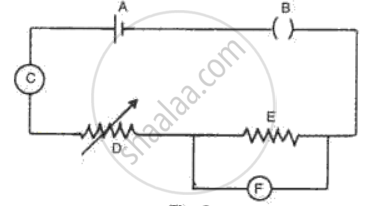Exercise | Q 2 | Page 210

What is the combined resistance of each of the networks between A and B shown in fig. ?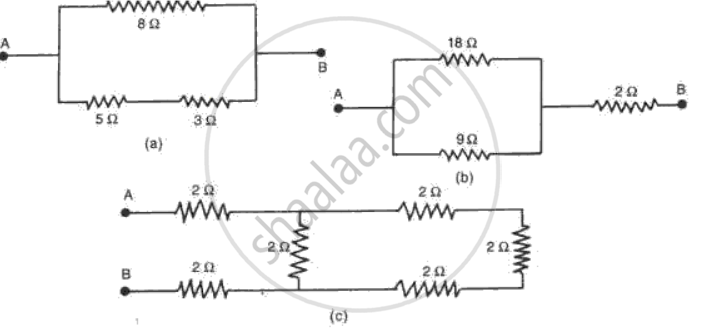Exercise | Q 3 | Page 210

A charge of 80 C flows in a conductor for 2 minutes.
(a) Calculate the current flowing through the conductor.
(b) If the current through a heater is 4 A what charge must be passing in 8 seconds?

Exercise | Q 4 | Page 210

The voltage across a 3Ω resistance is 6 V. How large is the current? What is
the resistance of a filament lamp when a voltage of 3 V across it causes a
current of 0.5 A?

Exercise | Q 5 | Page 210

Two resistors P and Q of the same material and length but of different thickness are connected in parallel to a battery. The cross- sectional area of P is twice that of Q. What is the ratio of
(a) The resistance of P to the resistance of Q?
(b) The current in P to the current in Q?

Exercise | Q 6 | Page 210

Show how would you connect three resistors, each of resistance 6 O so that
the combination has a resistance of(a) 9 Ω (b) 4 .Ω

Exercise | Q 7 | Page 210

A battery o f e.m.f. 3 Vis connected in series with an ammeter, a 10 Ω coil of wire and with a parallel combination o f resistance of 3Ω and 6 Ω. Draw a circuit diagram for the above arrangement. What is the
(a) Resistance of the parallel combination?
( c) Potential difference across the 3Ω resistor?
( d) Current flowing through each resistor?

Exercise [Page 211]

### Frank solutions for ICSE Class 10 Physics Part 2 Chapter 4 Current Electricity Exercise [Page 211]

Exercise | Q 1.1 | Page 211

Find the effective resistance in the following circuit diagrams (Fig.):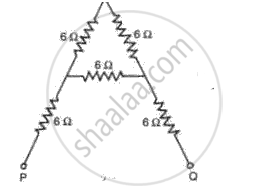Exercise | Q 1.2 | Page 211

Find the effective resistance in the following circuit diagrams (Fig.):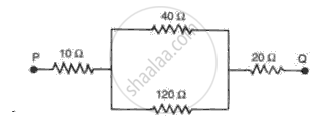Exercise | Q 1.3 | Page 211

Find the effective resistance in the following circuit diagrams (Fig.):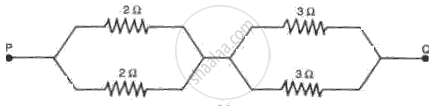Exercise | Q 1.4 | Page 211

Find the effective resistance in the following circuit diagrams (Fig.):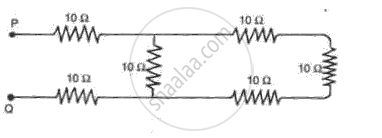Exercise | Q 1.5 | Page 211

Find the effective resistance in the following circuit diagrams (Fig.):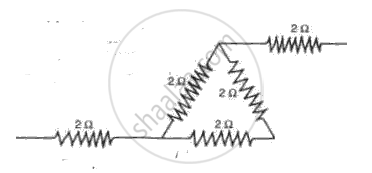Exercise | Q 2 | Page 211

Two cells each having an e.m.f. of 2 V and an internal resistance of 2Ω are
connected (a) In series, and ( b) In para 1le l as shown in fig. . What is the
current flowing through the cir cu it in each_ case?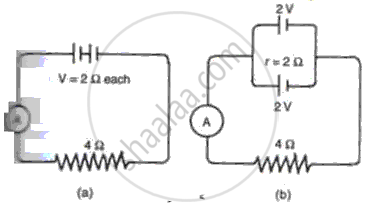Exercise | Q 3 | Page 211

In the circuit shown in fig. 6, find the reading of the ammeter A when the switch S is
(a) Opened.
(b) Closed.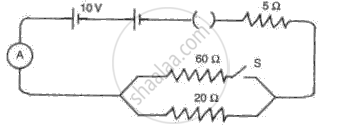Exercise | Q 4 | Page 211

A current of 0.3 A is flowing through a branch of 6 Ω resistors in a junction as shown in fig. . Calculate the
(a) P.d. across the junction XY,
(b) Current flowing through 9Ω and 24Ω
( c) P.d. across 24 Ω resistor, and
( d) e.m.f. of the cell.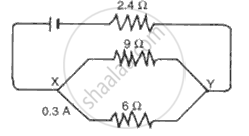Exercise [Page 212]

### Frank solutions for ICSE Class 10 Physics Part 2 Chapter 4 Current Electricity Exercise [Page 212]

Exercise | Q 1 | Page 212

The circuit diagram (Fig.) shows a battery of e.m.f. 6 volts and internal
resistance of 0.8 Ω oonnected in series. Find the
(a) Current reoorded by the ammeter,
(b) P.d. across the terminals of the resistor B,
( c) Current passing through each of the resistors B, C and D, and
( d) P.d. across the terminals of the battery.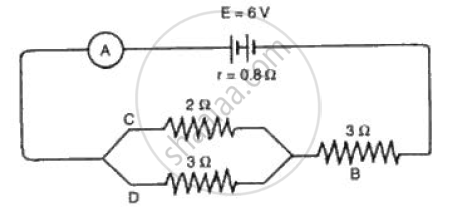Exercise | Q 2 | Page 212

Complete the following table. The first answer has been given as an example

 Quantity Unit (a) Electrical potential Volt ( b) Resistance ( c) Power ( d) Energy ( e) Resistivity Volt ........ .......... ............ ..............
Exercise | Q 3 | Page 212

The following appliances are to be used on 240 V supply. Calculate the current used by each and say which fuse, 2 amp, 5 amp or 13 amp should be incorporated with each, (i) A television rated at 150 W, (ii) An electric iron rated at 750 W, (iii) An immersion heater rated at 3000 W, (iv) A hair dryer rated at 500 W. How much will it cost to run the television set for 100 days for an average of 4 hours a day at 60 paise per unit?

Exercise | Q 4.1 | Page 212

What is the purpose of fuse in an electrical circuit?

Exercise | Q 4.2 | Page 212

What is the criteria for selecting a fuse wire?

Exercise | Q 4.3 | Page 212

If a fuse contains a 5 A fuse wire and the voltage is 240 V, what is the maximum power (wattage) which may be taken from the circuit?

Exercise | Q 4.4 | Page 212

What would be the danger involved in replacing a blown fuse with the one which would carry a large current?

Exercise | Q 5 | Page 212

An electric kettle has a heating element which is rated at 2 kW when connected to a 250 V electric supply. Calculate the
(a) Current which flows in the element when it is connected to a 250 V supply,
(b) Resistance of the filament,
(c) Heat produced in 1 minute by the element,
(d) Cost of running the kettle for 10 minutes a day for 30 days of the month, at the rate of Rs. 3.00 per unit.

Exercise | Q 6 | Page 212
Three fuses available are rated at 2 A, 10 A and 13 A. Which fuse would you chose for an electric wire rated at 3 kW, 250 V? Show how you arrived at your answer.
Exercise | Q 7 | Page 212

60 joules of heat was dissipated in a resistor when 20 C flowed for 5 s. Calculate:
(a) P.d. across the resistor,
(b) Resistance of the resistor, and
(c) Average power dissipated in the resistor.

Exercise | Q 8 | Page 212

Fig.  shows a 3 pin plug with the cover removed. The electric cable
connected to the plug contains 3 wires with colour coded insulation namely brown, blue and green.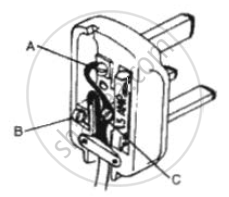1. Identify each of the colour coded wires by stating to which of the
terminals A, B or C in the diagram they should be connected.
2. Identify the terminal through which no current passes in normal
circumstances.
3. What is the purpose of the earthed wire connected to an electric
appliance like an electric heater? Describe how it works.
Exercise | Q 9 | Page 212
Explain the meaning of the term kilowatt hour.
Exercise [Page 213]

### Frank solutions for ICSE Class 10 Physics Part 2 Chapter 4 Current Electricity Exercise [Page 213]

Exercise | Q 1 | Page 213

In a house there are 6 bulbs of 100 W each used for 4 hours a day, 4 bulbs of 60 W each used for 8 hours a day, an immersion heater of 2.5 kW used for 1 hour per day, and an electric iron of 800 W used for 2 hours per day. Calculate the cost of monthly electric bill for the month of April at the rate of 80 p per unit.

Exercise | Q 2 | Page 213
Describe the construction and working of a filament lamp. List the material used and state why they are suitable for their purpose in this lamp.
Exercise | Q 3 | Page 213

Name the material and type of wires for the following:
(a) Connecting wires for lighting
(b) Fuse wire
(c) Heating elements
(d) Connecting wires of a power line
(e) Earthing elements

Exercise | Q 4 | Page 213
Two bulbs are marked 60 W, 220 V and 60 W, 110 V respectively. Calculate the ratio of their resistance.
Exercise | Q 5 | Page 213

An electric oven is rated as 1.5 kW, 250 V. If it is connected to a 250 V mains, calculate the
(a) Current drawn,
(b) Energy consumed in 20 hours,
(c) Cost of energy consumed in 20 hours at the rate of Re. 1 per unit.

Exercise | Q 6 | Page 213

An auto lamp is joined to a battery of e.m.f. 4 V and internal resistance 2.5Ω. A steady current of 0.5 A flows through the circuit. Calculate the
(a) Total energy provided by battery in 10 minutes,
(b) Heat dissipated in the bulb in 10 minutes.

Exercise | Q 7.1 | Page 213

What is meant by a ring circuit in house wiring?

Exercise | Q 7.2 | Page 213

What are the advantages of a parallel connection?

Exercise | Q 8.1 | Page 213

Explain with the help of a diagram how does 'short circuiting' occur in an electric kettle.

Exercise | Q 8.2 | Page 213

Out of the wires- live, neutral and earthing, on which line will you connect the fuse and the switch. Give reason for your answer.

Exercise | Q 9 | Page 213
What is meant by the term 'electromotive force' of a cell?
Exercise | Q 10 | Page 213

Explain, why is the p.d. between the terminals of a storage battery less when it is supplying current than when it is on open circuit. A battery of e.m.f. 10 volts and internal resistance 2.5 ohms has two resistances of 50 ohms each connected to it. Calculate the power dissipated in each resistance
(a) When they are in series,
(b) When they are in parallel.
In each case calculate the power dissipated in the battery.

Exercise | Q 11 | Page 213

Electrical appliances have voltage and power ratings as listed below. Which
has the larger working resistance?

 Appliances Voltage (V) Power (W) (a) Washing machine 250 3000 ( b) Television 240 160 (c)240 240 1500 ( d) Hair curler 250 20 ( e) Car head lamp 12 36
Exercise | Q 12 | Page 213

If the potential differences between two parts of a thundercloud is 10s V,
what is the amount of energy given up during the passage of 20 coulombs?

(a)2x10-7J

(b)200J

(c)Sx106 j

(d)2x 109J

(e)2x 1010 J

Exercise | Q 13 | Page 213

A 3 pin mains plug is fitted to the lead for a 1 kW electric kettle to be used on a 250 V A.C. supply. Which of the following statements is NOT correct?
(a) A 13 A fuse is the most appropriate value to use.
(b) The brown wire should be connected to the live side of the mains.

Exercise | Q 14.1 | Page 213

Name the principle on which a transformer works.

Exercise | Q 14.3 | Page 213

Can a transformer work when it is connected to a D.C. source? Give a reason.

Exercise | Q 14.4 | Page 213

Draw a simple labeled diagram of a step-down transformer.

Exercise | Q 14.5 | Page 213

Draw a simple labeled diagram of a step-up transformer.

Exercise | Q 15 | Page 213

The diagram 10 shows two coils X and Y. The coil X is connected to a battery S and a key K. The coil Y is connected to a galvanometer G.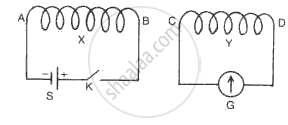When the key K is closed. State the polarity
(i)At the end of the coil X,
(ii)At the end C of the coil Y,
(iii)At the end C of the coil Y if the coil Y is (a) Moved towards the coil X, (b) Moved away from the coil X.

Exercise [Page 214]

### Frank solutions for ICSE Class 10 Physics Part 2 Chapter 4 Current Electricity Exercise [Page 214]

Exercise | Q 1 | Page 214

The following diagram shows a fixed coil of several turns connected to a
center zero galvanometer G and a magnet NS which can move.
(a) Describe the observation in the galvanometer if (i) The magnet is moved
rapidly in the direction of arrow, (ii ) The magnet is kept still after it has
moved into the coil, (iii ) The magnet~ then rapidly pulled out the coil.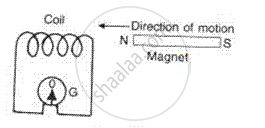(b) How would the observation alter if a more powerful magnet is used?

Exercise | Q 2.1 | Page 214

Tick (✓) the correct choice among the following :
(a) A strong short bar magnet is dropped into a solenoid connected to a
galvanometer as shown in fig . The galvanometer shows a deflection to the left when the current in the coil as seen from above is counter clockwise. Which one of the following shows the correct deflection of the galvanometer when the magnet is at posi tions A, Band C.

•  At A At B At C Left left left
•  At A At B At C Left zero zero
•  At A At B At C Left zero right
•  At A At B At C Left right left
•  At A At B At C Left right right
Exercise | Q 2.2 | Page 214

Tick (✓) the correct choice among the following :

Consider two cases of two parallel current carrying conductors. Current in
th e same direction and currents in th e opposi te directions will produce

• Attraction and repulsion respectively

• Repulsion and attraction respectively,

• Attraction in both cases

• Repulsion in both cases

• Osci llation in both cases

Exercise | Q 2.3 | Page 214

Tick (✓) the correct choice among the following :

Which of the following changes would enable a millimeter to be used as a
direct current ammeter reading upto 1 A?

•  connecting a large resistor in series

•  connecting a large resistor in parallel

•  connecting a small resistor in series

•  connecting a small resistor in parallel

Exercise | Q 2.4 | Page 214

Tick (✓) the correct choice among the following :

In fig .XY is at right angles to the magnetic field. The direction in
which XY should be moved to induce an electron flow in the direction Y to X as indicated by the arrow is

• Along the direction of XY

• Towards N and perpendicular to XY,

• Towards S and perpendicular to XY

• Upwards and perpendicular to XY

Exercise | Q 2.5 | Page 214

Tick (✓) the correct choice among the following :

There are 500 turns and 2000 turns in the primary and secondary coil of a
transformer respectively. If th e output voltage is 1000 V, how large is the
input voltage?

• 250 v

• 500 v

• 1000 v

• 2000 V

• 4000 V

Exercise | Q 3 | Page 214

Fig . shows the essent ial features of a battery operated bell. The hammer strikes the gong when the swi tch is closed . State and explain the effect of using the following material successively to form the core.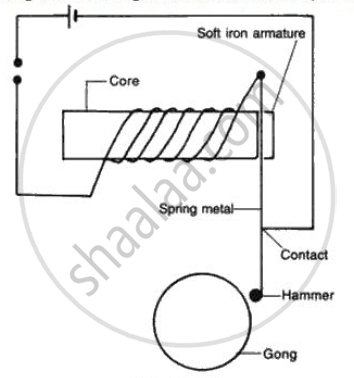(a) Plastic
(b) Steel
(c) Copper

Exercise [Page 215]

### Frank solutions for ICSE Class 10 Physics Part 2 Chapter 4 Current Electricity Exercise [Page 215]

Exercise | Q 1 | Page 215

A wire is passed through a piece of cardboard as shown in fig .. An electric current flows vertically down the wire and then flows back up. The two sections of the write are observed to repel each other.
(a) Wi th the aid of a diagram, describe how you would plot the magnetic field lines on the card .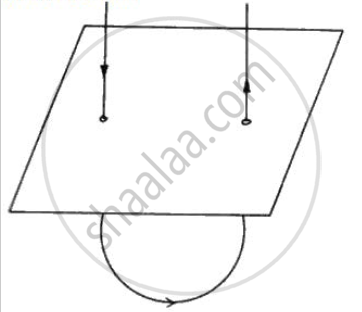(b) Sketch the magnetic field patterns around both the sections of the wire. Use your sketch to explain why the two sections repel each other.
(c) The repulsive force between the two sections is small. What changes could be made to increase it?

Exercise | Q 2 | Page 215

Draw and label the diagram of a simple D.C. motor.
(a) Explain the rotation of the coil, giving a reason for your answer.
(b) How can you reverse the direction of rotation of the armature?
(c) How can you increase the speed of rotation of the motor?

Exercise | Q 3 | Page 215

The diagram shows a coil connected to a center zero galvanometerG . The galvanometer shows a deflect ion to the right when the north pole N of a powerful magnet is moved to the right as shown .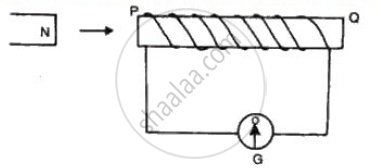(i) Explain, why the defelct ion occurs in the galvanometer.
(ii ) State whether th e current in the coil is cl ockwise or anticl ockwise
when viewed from the end P.
(iii ) State th e observation in G when the coil is moved away from north
pole N of the magnet keeping the magnet stationary.
(iv)State the observation in G when both the coil and the magnet are
moved to right at th e same speed .

Exercise | Q 4 | Page 215
A primary of 800 turns is connected to a 220 V A.C. supply and the secondary has 8 turns. What will be the output voltage?
Exercise | Q 5 | Page 215
How can you convert a moving coil galvanometer into ammeter and voltmeter?
Exercise | Q 6 | Page 215
Why is an ammeter always connected in series while the voltmeter always in parallel to a circuit across which voltage is to be measured?
Exercise | Q 7 | Page 215
Describe two experiments to illustrate the production of electric current by electromagnetic induction. State the laws which determine the direction and magnitude of the induced current.
Exercise [Page 216]

### Frank solutions for ICSE Class 10 Physics Part 2 Chapter 4 Current Electricity Exercise [Page 216]

Exercise | Q 1 | Page 216

The circuit diagram in fig  shows two coils of an insulated copper wire wound on a cardboard tube. G is a center zero galvanometer.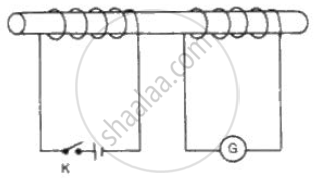(a) Describe what will happen when th e swi tch K is cl osed for severa l
seconds and then opened again .
(b) What will be the effect of repeating the experiment with an iron placed in the tube?

Exercise | Q 2 | Page 216

Fig . shows the core of a transformer and i ts input and output connections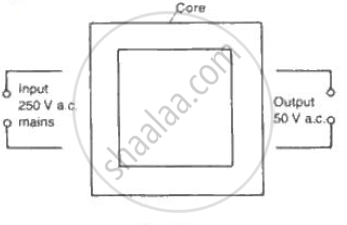State the material used for the core and describe th e structure of the core.
(a) Use the values given in the diagram to calculate the turns ratio for the transformer and connections, labeling all parts of the diagram .
(b) If a current of 2 A is taken from th e output, calculate th e current in the input circuit (Assume the transformer to be ideal ).

Exercise | Q 3 | Page 216

Fig. shows a simple form of an A.C. generator.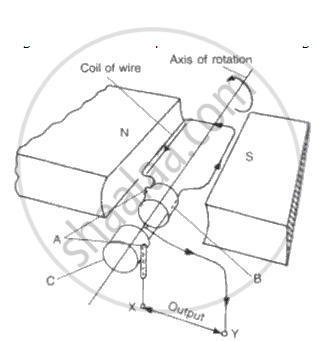(a) Name the parts labeled A and B.
(b) What would be the effect of doubling the number of turns on the coil if the speed of rotation remains unchanged?
(c) Which of the output terminals is positive if the coil is rotating in the
direction shown in the diagram (anticlockwise)?
( d ) What is the position of the rotating coil when p.d. across its ends is zero? Explain why p.d. is zero when the coil is at this position .
(e) Sketch a graph showing how the p.d. across the ends of the rotating coil varies with time for an A.C. dynamo.
( f) On th e same sheet of paper and vertically below the first graph using the same time scale, sketch graphs to show the effect of
(i) Doubling the speed of rotation and at the same time keeping
the field and the number of turns constant,
(ii ) Doubling the number of turns on the coil and at the same time
doubling the speed of rotation of the coil, keeping th e speed
constant.

Exercise | Q 4 | Page 216

Name the type of transformer shown in fig . What changes will you observe regarding the brightness of the lamp, if
(a) The no. of turns in the secondary is doubled?
(b) The part X of the transformer is removed?
( c) The core of the transformer is made out of copper?
(d ) The A.C. supply is replaced by D.C.?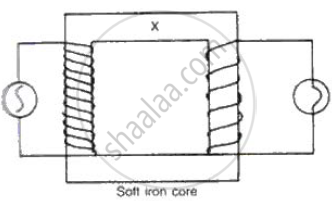Exercise [Page 217]

### Frank solutions for ICSE Class 10 Physics Part 2 Chapter 4 Current Electricity Exercise [Page 217]

Exercise | Q 1 | Page 217

A transformer has 400 turns in the primary winding and 10 turns in the secondary winding. The primary e.m.f. is 250 V and the primary current is 2.0 A. calculate:
(a) The secondary voltage,
(b) The secondary current, assuming 100% efficiency.

Exercise | Q 2.1 | Page 217

Fill in the blanks by writing (i) Only soft iron, (ii) Only steel, (iii) Both soft-iron and steel for the material of core and/or magnet.

Electric bell ______.

Exercise | Q 2.2 | Page 217

Fill in the blanks by writing (i) Only soft iron, (ii) Only steel, (iii) Both soft-iron and steel for the material of core and/or magnet.

Electromagnet ______.

Exercise | Q 2.3 | Page 217

Fill in the blanks by writing (i) Only soft iron, (ii) Only steel, (iii) Both soft-iron and steel for the material of core and/or magnet.

D.C. motor ______.

Exercise | Q 2.4 | Page 217

Fill in the blanks by writing (i) Only soft iron, (ii) Only steel, (iii) Both soft-iron and steel for the material of core and/or magnet.

A. C. generator______.

Exercise | Q 2.5 | Page 217

Fill in the blanks by writing (i) Only soft iron, (ii) Only steel, (iii) Both soft-iron and steel for the material of core and/or magnet.

Transformer______.

Exercise | Q 3 | Page 217

The circuit diagram (Fig .) shows three resistors 2 Ω, 4 Ω and RΩ connected to a battery of e.m.f. 2 V and internal resistance 3 Ω. A main current of 0 .25
A flows through the circuit.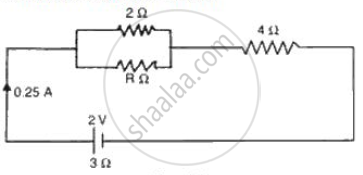(i ) What is the p.d . across the 4 Ω resistor?
(ii ) Calculate the p.d . across the in ternal resistance of the cell.
(iii ) What is the p.d . across the R Ω or 2 Ω resistors?
(iv) Calculate the value of R.

Exercise | Q 4 | Page 217
Transformers are usually designed so that their efficiency is as close to 100% as possible. Describe two features in transformer design which help to achieve high efficiency?
Exercise | Q 5 | Page 217

A cell supplies a current of 1.2 A through two 2Ω resistors connected in parallel. When the resistors are connected in series, if supplies a current of 0.4 A. Calculate the internal resistance and e.m.f of the cell.

Exercise | Q 6 | Page 217

Complete the following diagram of a transformer and name the parts labeled A and B. Name the part you have drawn to complete the diagram . What is the material of this part? In this transformer a step-up or step-down? Why?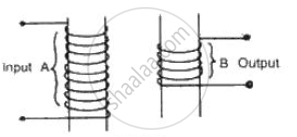Exercise | Q 7 | Page 217
Why do you mean by turns ratio of a transformer? Can it work with D.C.?

## Chapter 4: Current Electricity

Exercise 4.1ExerciseExercise 4.2Exercise 4.3## Frank solutions for ICSE Class 10 Physics Part 2 chapter 4 - Current Electricity

Frank solutions for ICSE Class 10 Physics Part 2 chapter 4 (Current Electricity) include all questions with solution and detail explanation. This will clear students doubts about any question and improve application skills while preparing for board exams. The detailed, step-by-step solutions will help you understand the concepts better and clear your confusions, if any. Shaalaa.com has the CISCE ICSE Class 10 Physics Part 2 solutions in a manner that help students grasp basic concepts better and faster.

Further, we at Shaalaa.com provide such solutions so that students can prepare for written exams. Frank textbook solutions can be a core help for self-study and acts as a perfect self-help guidance for students.

Concepts covered in ICSE Class 10 Physics Part 2 chapter 4 Current Electricity are Ohm’s Law, Concepts of Emf, Resistance of a System of Resistors - Resistors in Parallel, Electric Potential Difference, Concepts of Pd (V), Current (I), Resistance (R) and Charge (Q)., Internal Resistance, Resistance of a System of Resistors - Resistors in Series, Concept of Current Electricity, Concept of Current Electricity Numericals, Ac Generator, Household Circuits - Colour Coding of Wires, Household Circuits - Three-pin Plugs, Household Circuits - Safety Precautions, Household Circuits - Earthing;, Household Circuits - Fuses, Household Circuits - Switches, Household Circuits – Main Circuit, Electrical Energy and Power, Concept of Electrical Power and Household Circuits Numericals, Electric Power, Ac Generator, Concept of Current Electricity, Resistance of a System of Resistors - Resistors in Series, Concept of Transformer, Electromagnetic Induction, Magnetic Effect of a Current, Fleming’s Left Hand Rule, Ac Generator, Fleming’S Right Hand Rule, Concept of Electro Magnetism, Lenz’S Law and Conservation of Energy, Eddy Currents.

Using Frank Class 10 solutions Current Electricity exercise by students are an easy way to prepare for the exams, as they involve solutions arranged chapter-wise also page wise. The questions involved in Frank Solutions are important questions that can be asked in the final exam. Maximum students of CISCE Class 10 prefer Frank Textbook Solutions to score more in exam.

Get the free view of chapter 4 Current Electricity Class 10 extra questions for ICSE Class 10 Physics Part 2 and can use Shaalaa.com to keep it handy for your exam preparation